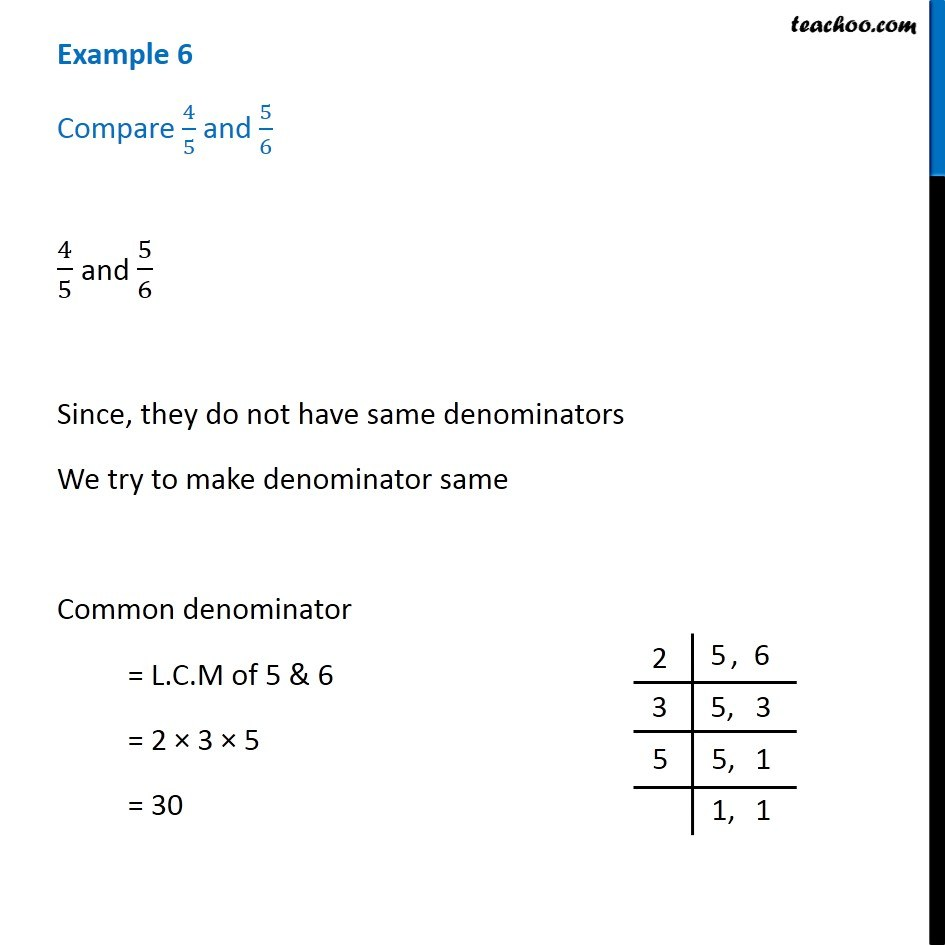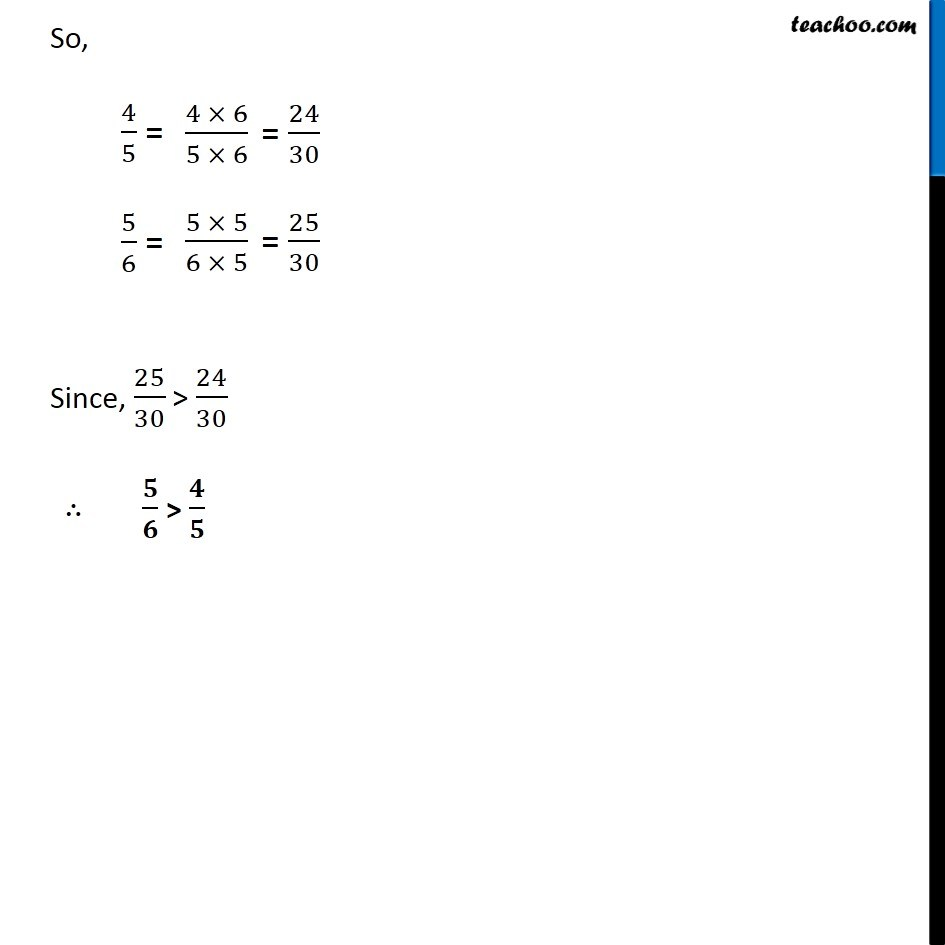1. Chapter 7 Class 6 Fractions
2. Serial order wise
3. Examples

Transcript

Example 6 Compare 4/5 and 5/6 4/5 and 5/6 Since, they do not have same denominators We try to make denominator same Common denominator = L.C.M of 5 & 6 = 2 × 3 × 5 = 30 So, 4/5 = 5/6 = Since, 25/30 > 24/30 ∴ 𝟓/𝟔 > 𝟒/𝟓

Examples

Chapter 7 Class 6 Fractions
Serial order wise